NCERT Solutions - Long And Short

# Long And Short NCERT Solutions - Mathematics for Class 4: NCERT

Page No - 14

Try This
Ques 1:
(a) Make her right arm 1 cm longer than the left arm.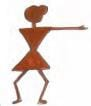(b) Draw a cup 1 cm shorter than this cup.(c) Draw a broom half as long as this broom.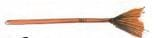(d) Draw another hair of double the length.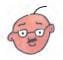Ans:
(a)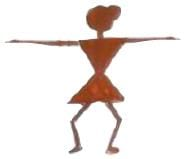(b)(c)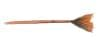(d)Page No - 15

How Tall Have You Grown?Ques 1:

(a) Do you remember that in Class 3 you measured your height?
(b) Do you think you have grown taller?
(c) How much? ____________ (cm)
(d) Have your friends also grown taller?
Ans:
(a) yes
(b) Yes I grew taller

(d) Yes, most of them.
HintThese are the sample answer for reference. Answer may be vary from according to students.

Ques 2: Jhumpa once read a list of the tallest people in the world. One of them was 272 cm tall! That is just double of Jhumpa’s height. How tall is Jhumpa? ____________ cm.
Ans:
272 ÷ 2 = 131 cm

The Long and Short of Your Family!(a) Who is the tallest in your family? _____
Ans: My father is the tallest in my family. His height is 173 cm.
(b) Who is the shortest in your family? _____
Ans: My younger brother is the shortest in my family. His height is 96 cm.

(c) What is the difference between their heights? ____
Ans:
What is the difference between their heights?

Page No - 16

Race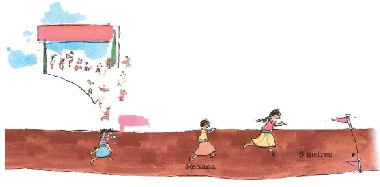Ques 1: This is a 100 metre race for girls. Arundhati is nearest the finishing line. She is about 6 metres from it.
Behind her is Rehana. Konkana and Uma are running behind Rehana. Look at the picture. To answer the questions below choose from these distances:
(i) 3 metres
(ii) 6 metres
(iii) 10 metres
(iv) 15 metres

(a) How far is Rehana from Arundhati? ______________
(b) How far ahead is Rehana from Konkana and Uma? ___________
(c) How far are Konkana and Uma from the finishing line? __________
Ans:
(a) By observing the figure, we see that the distance between Rehana and Arundhati is smaller than the distance of Rehana from Konkana and Uma. So, the distance between Rehana and Arundhati is 3 metres.
(b) By observing the figure, we see that the distance of Rehana from Konkana and Uma is double the distance between Rehana and Arundhati. Thus, Rehana is 6 metres ahead of Konkana and Uma.
(c) Since Rehana is 6 metres ahead of Konkana and Uma, Arundhati is 3 metres ahead of Rehana and Arundhati is 6 metres behind the finishing line.
∴ Distance of the finishing line from Konkana and Uma = 6 m + 3 m + 6 m = 15 m Thus, Konkana and Uma are 15 metres far away from the finishing line.

Ques 2:  Have you heard about a 1500 m or 3000 m race? (You remember that 1000 metres make 1 kilometre and 500 metres make half a kilometre.)
So you can say
(a) In a 1500 metres race people run _________ km.
(b) In a 3000 metres race people run _________ km.
Ans:We know 1 km = 1000 m

Therefore, Half km = 500 m
Now, 1500 m = 1000 m + 500 m = (1000 ÷ 1000) km + (500 ÷ 1000) m
= 1 km + Half km = 1 and a half kilometres
(a) In a 1500 metres race people run 1 and a half km
3000 m = (3000 ÷ 1000) km = 3 km.
(b) In a 3000 metres race people run 3 km

Page No - 17

Ques 1: Have you heard about marathon races in which people have to run about 40 kilometres? People run marathons on roads because the track of a stadium is only 400 metres.
(a) 10 rounds of a stadium track = _______ km

(b) So, if you run a marathon on a stadium track, you will have to complete _____________ rounds !
Ans: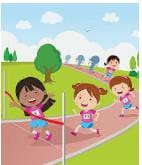We know 1000 m = 1 km
Length of the stadium track = 400 m
Distance covered in 1 round = 400 m
∴ Distance covered in 10 rounds = (10×400) m = 4000 m
= (4000÷1000) km = 4 km
Therefore,
(a) 10 rounds of a stadium track = 4 km
Total length of the marathon race = 40 km = (40×1000) m = 40000 m
Distance covered in 1 round = 400 m
Number of rounds to be taken to complete the race = (40000÷400) = 100
(b) So, if you run a marathon on a stadium track, you will have to complete 100 rounds !

Long Jump
Ques 2: Dhanu has the longest jump of 3 metres 40 cm. Gurjeet is second. His jump is 20 cm less than Dhanu’s. Gopi comes third. His jump is only 5 cm less than Gurjeet’s jump.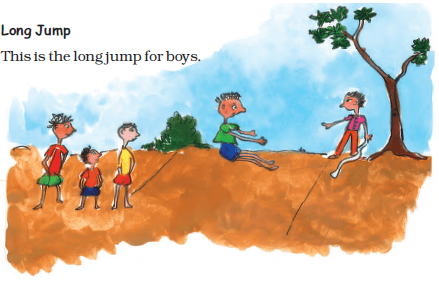(a) How long are Gurjeet’s and Gopi’s jumps?______________________________
(b) Try and see how far you can jump.
(c) How far can you throw a ball? ____________ metres.
(d) Look for a big ball, like a football or volleyball. How far can you kick it? ___________
Ans:
(a) We know 1 m = 100 cm
Length of Dhanu’s jump = 3 m 40 cm = (3×100) cm + 40 cm = 300 cm + 40 cm = 340 cm
Since Gurjeet’s jump is 20 cm less than that Dhanu’s jump, we will subtract 20 cm from 340 cm to find the length of Gurjeet’s jump.
340cm - 20cm = 320cm
Now, Length of Gurjeet’s jump = 320 cm = 300 cm + 20 cm = (300÷100) m + 20 cm = 3 m + 20 cm = 3 m 20 cm
Therefore, Gurjeet’s jump is 3 m 20 cm long.
Since Gopi’s jump is 5 cm less than Gurjeet’s jump, we will subtract 5 cm from 320 cm to find the length of Gopi’s jump 320cm - 5cm = 315cm.
Now, Length of Gopi’s jump = 315 cm = 300 cm + 15 cm = (300 ÷ 100) m + 15 cm = 3 m + 15 cm = 3 m 15 cm
Therefore, Gopi’s jump is 3 m 15 cm long.
(b) I can jump one and half metre.
Hint: The answer may vary from student to student, based on his/her observation. It is highly recommended that the students prepare the answer on their own.
(c) I can throw a ball to a distance of 5 metres.
HintThe answer may vary from student to student, based on his/her observation. It is highly recommended that the students prepare the answer on their own.
(d) I can kick a ball to a distance of 25 metres
HintThe answer may vary from student to student, based on his/her observation. It is highly recommended that the students prepare the answer on
their own

Page No - 18

Ques 1: Here are the Indian Records and World Records from some jumps.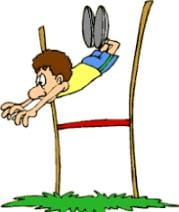Sports World Record Indian Record High Jump (Men) Javier S. (2 m 45 cm) Chandra Pal (2 m 17 cm) Long Jump (Men) Mike P. (8 m 95 cm) Amrit Pal (8 m 8 cm) High Jump (Women) Stefka K. (2 m 9 cm) Bobby A. (1 m 91 cm) Long jump (Women) Galina C. (7 m 52 cm) Anju G. (6 m 83 cm)

Find out from the table-
(1) How many centimetres more should Chandra Pal jump to equal the Men’s World Record for hih jump?
Ans: We know 1 m = 100 cm
Men’s world record for high jump = 2 m 45 cm = 2 m + 45 cm = (2 ×100) cm + 45 cm = 200 cm + 45 cm = 245 cm
High jump record of Chandra Pal = 2 m 17 cm = 2 m + 17 cm = (2×100) cm + 17 cm = 200 cm + 17 cm = 217 cm Now, we will subtract 217 cm from 245 cm.
245 cm - 217 cm = 28 cm
Thus, Chandra Pal should jump 28 cm more to equal the men’s world record for high jump.

(2) How many centimetres higher should Bobby A. jump to reach 2 metres?
Remember that 1 m = 100 cm Half metre = ?

Ans: We know 1 m = 100 cm
Thus, 2 m = (2×100) cm = 200 cm
High jump of Bobby A. = 1 m 91 cm = 1 m + 91 cm = 100 cm + 91 cm = 191 cm
Now, 200 cm − 191 cm = 9 cm
Thus, Bobby A. should jump 9 centimetres higher to reach 2 metres.

(3) Galina’s long jump is nearly
(a) 7 metres
(b) 7 and a half metres
(c) 8 metres
Ans:
Correct: (b)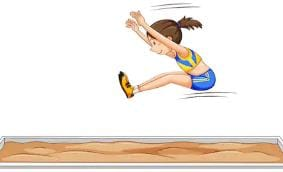Galina’s long jump record is 7 m 52 cm
Hence, it is nearly 7 and half metres,
Therefore option (b) is correct

(4) Look at the Women’s World Records. What is the difference between the longest jump and the highest jump?
Ans: We know 1 m = 100 cm
Long jump of Galina C.
= 7 m 52 cm = (7 ×100) cm + 52 cm = 700 cm + 52 cm = 752 cm
High jump of Stefka K.
= 2 m 9 cm = (2×100) cm + 9 cm = 200 cm + 9 cm = 209 cm
Now, to find the difference between the longest jump and the highest jump, we have to subtract 209 cm from 752 cm.
752 cm - 209 cm = 543 cm
∴ Difference between the longest jump and the highest jump = 543 cm = 500 cm + 43 cm = (500÷100) m + 43 cm = 5 m + 43 cm = 5 m 43 cm

(5) If Mike P. could jump ________ centimetres longer, his jump would be full 9 metres.
Ans:
We know 1 m = 100 cm
Now, 9 m = (9×100) cm = 900 cm
High jump of Mike P. = 8 m 95 cm = 8 m + 95 cm = (8×100) cm + 95 cm = 800 cm + 95 cm = 895 cm
Now, 900 cm − 895 cm = 5 cm
If Mike P. could jump 5 centimetres longer, his jump would be full 9 metres.

(6) Whose high jump is very close to two and half metres?
(a) Stefka K.
(b) Chandra Pal
(c) Javier S.
(d) Bobby A.

Ans: We know 1 m = 100 cm 0.5 m = 50 cm
Two and half metres = 2 m + Half metre = (2×100) cm + 50 cm = 200 cm + 50 cm = 250 cm
High jump of Stefka K. = 2 m 9 cm = (2 ×100) cm + 9 cm = 200 cm + 9 cm = 209 cm
High jump of Chandra Pal = 2 m 17 cm = (2 ×100) cm + 17 cm = 200 cm + 17 cm = 217 cm
High jump of Javier S. = 2 m 45 cm = (2 ×100) cm + 45 cm = 200 cm + 45 cm = 245 cm
High jump of Bobby A. = 1 m 91 cm = 100 cm + 91 cm = 191 cm
We can see, 245 cm is very close to 250 cm.
Thus, the high jump of Javier S. is very close to two and half metres.

Page No - 19

Running Exercise

Ques 1: The doctor has told Devi Prasad to run 2 km every day to stay fit. He took one round of this field. How far did he run? The field was very far from his home. So he chose a park nearby. The boundary of the park was about 400 metres long.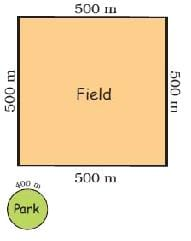(a) How many rounds of the park must Devi Prasad run to complete 2 km?
(b) One day the weather was very good and a cool breeze was blowing. He felt so good that he kept jogging till he got tired after 8 rounds. That day he ran ___________ km and __________ metres !
Ans:
(a) Devi Prasad took 1 round of the square field whose each side was 500 m long. Distance covered to take 1 round of the field = (500 + 500 + 500 + 500) m = 2000 m
We know 1000 m = 1 km Thus, 2000 m = 2 km.
So, Devi Prasad ran 2 km to take one round of the field as advised by the doctor. Length of the boundary of the park = 400 m.
Number of rounds of the park taken by Devi Prasad to run 400 m = 1
Number of rounds of the park taken by Devi Prasad to run 2000 m = 2000 ÷ 400 = 5
We know 2000 m = 2 km
So, Devi Prasad must take 5 rounds in order to complete 2 km.
(b) Distance covered by Devi Prasad to take 1 round of the park = 400 m
∴ Distance covered by Devi Prasad to take 8 rounds of the park =400 m × 8 = 3200 m
We know 1000 m = 1 km
∴ 3200 m = 3000 m + 200 m
3200 m = 3 km + 200 m
3200 m = 3 km 200 m
Thus, Devi Prasad ran 3 km 200 m to take 8 rounds of the park.

Page No - 20

From Kozhikode to Thalassery
Ques 1:  Subodh is going to Kozhikode which is 24 kilometres (km) away. Manjani is going to Thalassery which is 46 km away in the opposite direction.
How far is Kozhikode from Thalassery? ____________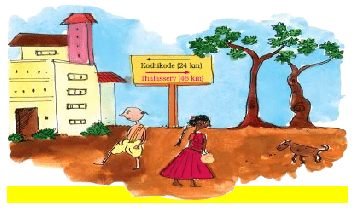Ans: Suppose Subodh and Manjani start from the same point.
Distance of Kozhikode from the starting point = 24 km
Distance of Thalassery from the starting point = 46 km
Subodh is going to Kozhikode and Manjani is going to Thalassery. Both of them are travelling in opposite directions.
∴ Distance between Kozhikode and Thalassery = (24 + 46) km = 70 km.
Thus, Kozhikode is 70 km away from Thalassery.

How Far is Your Home from School?
Ques 2:  Momun comes to school from very far. He first walks about 400 metres to the pond. With slippers in his hands, he then walks 150 metres through the pond. Next he runs across the 350 metres wide green field. Then he carefully crosses the 40 metres wide road to reach his school.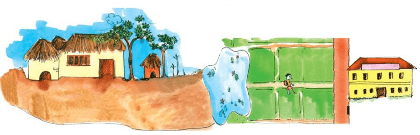How much does Momun walk every day to reach school? _________
Is it more than 1 km? ________
Ans: Distance covered by Momun to reach the pond = 400 m
Distance covered by Momun while walking through the pond = 150 m
Distance covered by Momun while running across the green field = 350 m Distance covered by Momun to cross the road = 40 m
Total distance covered by Momun to reach the school = (400 + 150 + 350 + 40) m = 940 m
Therefore, Momun walks 940 m every day to reach the school.
We know 1 km = 1000 m. Also, 940 m is less than 1000 m.
Thus, the total distance travelled by Momun to reach the school every day is not more than 1 km.

Page No - 21

Ques 1: Find out how far your friends live from school and fill the table. Write in metres or kilometres.

 Friend’s name Distance of home from school

(a) Who among you lives nearest to the school? _________ .
(b) Who lives farthest from the school? ___________.
(c) How many children live less than 1 kilometre away from your school? __________

(d) Is there anyone who lives more than 5 km away from the school? How do they come to school? ________________
Ans:

 Friend’s name Distance of home from school Akshay 6 km Varun 4 km Shikhar 9 km Brijendra 500 m Pooja 600 m Gaurav 7 km

(a) Brijendra lives nearest to the school.
(b) Shikhar lives farthest from the school.
(c) There are 2 children—Brijendra and Pooja—who live less than 1 kilometre away from the school.
(d) There are 3 children—Shikhar, Akshay and Gaurav—who live more than 5 kilometres away from the school. They come to school by school bus.

Hint: The answer may vary from student to student, based on his/her experience. The answer provided here is for reference only.

The document Long And Short NCERT Solutions | Mathematics for Class 4: NCERT is a part of the Class 4 Course Mathematics for Class 4: NCERT.
All you need of Class 4 at this link: Class 4

## Mathematics for Class 4: NCERT

25 videos|84 docs|41 tests

## FAQs on Long And Short NCERT Solutions - Mathematics for Class 4: NCERT

 1. What is the concept of long and short in the context of NCERT solutions?Ans. In the context of NCERT solutions, the concept of long and short refers to the length of the answers provided for a particular question. A long answer generally requires more detailed explanations and examples, while a short answer can be more concise and to the point.
 2. Are long answers always better than short answers in NCERT solutions?Ans. No, long answers are not always better than short answers in NCERT solutions. The length of the answer depends on the complexity of the question and the level of detail required to adequately address it. Sometimes, a short answer can be sufficient if it covers all the key points and concepts accurately.
 3. How can I determine whether a question in NCERT solutions requires a long or a short answer?Ans. To determine whether a question in NCERT solutions requires a long or a short answer, it is essential to carefully read the question and analyze its requirements. If the question asks for specific examples, explanations, or detailed analysis, a long answer may be necessary. On the other hand, if the question is straightforward and only requires a brief response, a short answer would suffice.
 4. Are there any specific guidelines provided by NCERT for writing long and short answers?Ans. Yes, NCERT provides guidelines for writing long and short answers in their solutions. These guidelines emphasize the importance of providing accurate information, using appropriate language, and organizing the answer in a logical and coherent manner. For long answers, students are encouraged to include relevant examples, diagrams, and step-by-step explanations, whereas short answers should be concise yet comprehensive.
 5. How can I ensure that my long and short answers in NCERT solutions are effective and score well in exams?## Mathematics for Class 4: NCERT

25 videos|84 docs|41 testsExplore Courses for Class 4 examSignup to see your scores go up within 7 days! Learn & Practice with 1000+ FREE Notes, Videos & Tests.
10M+ students study on EduRev
Track your progress, build streaks, highlight & save important lessons and more!
Related Searches

,

,

,

,

,

,

,

,

,

,

,

,

,

,

,

,

,

,

,

,

,

;